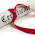# Placement Paper - HCL - C Programming

1. Which of the following about the following two declaration is true
i ) int *F()
ii) int (*F)()
Choice :
a) Both are identical
b) The first is a correct declaration and the second is wrong
c) The first declaration is a function returning a pointer to an integer and the second is a pointer to function returning int
d) Both are different ways of declaring pointer to a function
2. What are the values printed by the following program?
#define dprint(expr) printf(#expr "=%d\n",expr)
main()
{
int x=7;
int y=3;
dprintf(x/y);
}
Choice:
a) #2 = 2 b) expr=2 c) x/y=2 d) none

3. What is th output of the following program?
int x= 0x65;
main()
{
char x;
printf("%d\n",x)
}
a) compilation error     b) 'A'     c) 65       d) unidentified
4. What is the output of the following program
main()
{
int a=10;
int b=6;
if(a=3)
b++;
printf("%d %d\n",a,b++);
}
a) 10,6 b)10,7 c) 3,6 d) 3,7 e) none

5. What can be said of the following program?
main()
{
enum Months {JAN =1,FEB,MAR,APR};
Months X = JAN;
if(X==1)
{
printf("Jan is the first month");
}
}
a) Does not print anything
b) Prints : Jan is the first month
c) Generates compilation error
d) Results in runtime error

6. What is the output of the following program?
main()
{
int l=6;
switch(l)
{ default : l+=2;
case 4: l=4;
case 5: l++;
break;
}
printf("%d",l);
}
a)8 b)6 c)5 d)4 e)none

7. What is the output of the following program?
main()
{
int x=20;
int y=10;
swap(x,y);
printf("%d %d",y,x+2);
}
swap(int x,int y)
{
int temp;
temp =x;
x=y;
y=temp;
}
a)10,20 b) 20,12 c) 22,10 d)10,22 e)none

8. What is the size of the following union. Assume that the size of int =2, size of float =4 and size of
char =1.
Union Tag{
int a;
flaot b;
char c;
};
a)2 b)4 c)1 d) 7

Disclaimer: These are questions/Answers posted by various candidates over years, TutionCentral(TC) does not assume any legal liability or responsibility for the accuracy, completeness or usefulness of the information. Information provided in the site is solely intended for general guidance purpose and assumes the reader of the page full responsibility of use.